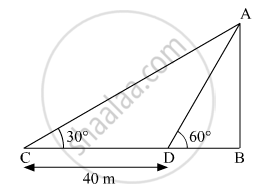# The shadow of a tower standing on level ground is found to be 40 m longer when the Sun's altitude is 30° than when it was 60°. Find the height of the tower. (Given sqrt3= 1.732) - Mathematics

Short Note

The shadow of a tower standing on level ground is found to be 40 m longer when the Sun's altitude is 30° than when it was 60°. Find the height of the tower. (Given sqrt3 = 1.732)

#### SolutionLet AB is the tower and BD is the length of the shadow when the sun's altitude is 60°°.
Let, AB be x m and BD be y m.
So, CB = (40 + y) m
Now, we have two right-angled triangles ΔABD and ΔABC
In ABD

tan 60^@ = "AB"/"BD"

sqrt3 = "x"/"y"

In ABC

tan 30^@ = "AB"/"BD"

1/sqrt3 = "x"/("y"+40) ......(1)

We have x = y sqrt3

substituting the value in (1)

("y"sqrt3)sqrt3 = "y" + 40

⇒ 3y = y + 40

⇒ y = 20

⇒ "x" = 20 sqrt3"m" = 34.64"m"

Hence, the height of the tower is 20 sqrt3"m".

Concept: Heights and Distances
Is there an error in this question or solution?